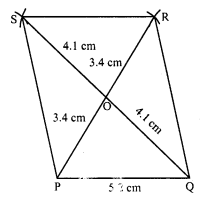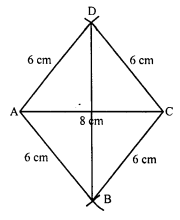# RD Sharma Class 8 Solutions Chapter 18 Practical Geometry Ex 18.1

In this chapter, we provide RD Sharma Class 8 Solutions Chapter 18 Practical Geometry Ex 18.1 for English medium students, Which will very helpful for every student in their exams. Students can download the latest RD Sharma Class 8 Solutions Chapter 18 Practical Geometry Ex 18.1 Maths pdf, free RD Sharma Class 8 Solutions Chapter 18 Practical Geometry Ex 18.1 book pdf download. Now you will get step by step solution to each question.

### RD Sharma Solutions for Class 8 Chapter 18 Practical Geometry Ex 18.1Download PDF

Question 1.
Construct a quadrilateral ABCD in which AB = 4.4 cm, BC = 4 cm, CD = 6.4 cm, DA = 3.8 cm and BD = 6.6 cm.
Solution:
Steps of construction :
(i) Draw a line segment AB = 4.4 cm.(ii) With centre A and radius 3.8 cm and with centre B and radius 6.6 cm, draw arcs intersecting each other at D.
(iii) With centre B and radius 4 cm, and with centre D and radius 6.4 cm, draw arcs intersecting each other at C on the other side of BD.
(iv) Join AD, BD, BC and DC.
The ABCD is the required quadrilateral.

Question 2.
Construct a quadrilateral ABCD such that AB = BC = 5.5 cm, CD = 4 cm, DA = 6.3 cm and AC = 9.4 cm. Measure BD.
Solution:
(i) Draw a line segment AC = 9.4 cm.
(ii) With centre A and C and radius 5.5 cm, draw arcs intersecting each other at B.
(iii) Join AB and CB.
(iv) Again with centre A and radius 6.3 cm, and with centre C and radius 4 cm, draw arcs intersecting each other at D below the line segment AC.Then ABCD is the required quadrilateral. On measuring BD, it is 5 cm.

Question 3.
Construct a quadrilateral XYZW in which XY = 5 cm, YZ = 6 cm, ZW = 7 cm, WX = 3 cm and XZ = 9 cm.
Solution:
Steps of construction :
(i) Draw a line segment XZ = 9 cm.
(ii) With centre X and radius 3 cm and with centre Z and radius 7 cm, draw arcs intersecting each other at W.(iii) Join XW and ZW.
(iv) Again with centre X and radius 5 cm and with centre Z and radius 6 cm, draw arcs, intersecting each other at Y below the line segment XZ.
(v) Join XY and ZY.
Then XYZW is the required quadrilateral

Question 4.
Construct a parallelogram PQRS such that PQ = 5.2 cm, PR = 6.8 cm and QS = 8.2 cm.
Solution:
Steps of construction:
In a parallelogram, diagonals bisect each other. Now
(i) Draw a line segment PQ = 5.2 cm.
(ii) With centre P and radius 3.4 cm (12 of PR) and with centre Q and radius 4.1 cm (12 of QS) draw arcs intersecting each other at O.(iii) Join PQ and QO and produced them to R and S respectively such that PO = OR and QO = OS.
(iv) Join PS, SR and RQ.
Then PQRS is the required parallelogram

Question 5.
Construct a rhombus with side 6 cm and one diagonal 8 cm. Measure the other diagonal.
Solution:
Steps of construction :
Sides of a rhombus are equal.
(i) Draw a line segment AC = 8 cm.(ii) With centres A and C and radius 6 cm, draw two arcs above the line segment AC and two below the line segment AC, intersecting each other at D and B respectively.
(iii) Join AB, AD, BC and CD.
Then ABCD is the required rhombus.
JoinBD.
On measuring BD, it is approximately 9 cm

Question 6.
Construct a kite ABCD in which AB = 4 cm, BC = 4.9 cm and AC = 7.2 cm.
Solution:
Steps of construction :
(i) Draw a line segment AC = 7.2 cm.(ii) With centre A and radius 4 cm draw an arc.
(iii) With centre C and radius 4.9 cm, draw another arc which intersects the first arc at B and D.
(iv) Join AB, BC, CD and DA.
Then ABCD is the required kite.

Question 7.
Construct, if possible, a quadrilateral ABCD given, AB = 6 cm BC = 3.7 cm, CD = 5.7 cm, AD = 5.5 cm and BD = 6.1 cm. Give reasons for not being able to construct, if you cannot.
Solution:
Steps of construction :
(i) Draw a line segment BD = 6.1 cm.
(ii) With centre B and radius 6 cm and with centre D and radius 5.5 cm, draw arcs intersecting at A.
(iv) Again with centre B and radius 3.7 cm and with centre D and radius 5.7 cm, draw two arcs intersecting each other at C below the BD.(v) Join BC and DC.
Then ABCD is the required quadrilateral.

Question 8.
Construct, if possible a quadrilateral ABCD in which AB = 6 cm, BC = 7 cm, CD = 3 cm, AD = 5.5. cm and AC = 11 cm. Give reasons for not being able to construct, if you cannot.
Solution:
Steps of construction:
It is not possible to construct this quadrilateral ABCD because
AD + DC = 5.5 cm + 3 cm = 8.5 cm
and AC = 11 cm
But we know that in a triangle,
Sum of two sides is always greater than its third side.

All Chapter RD Sharma Solutions For Class 8 Maths

—————————————————————————–

All Subject NCERT Exemplar Problems Solutions For Class 8

All Subject NCERT Solutions For Class 8

*************************************************

I think you got complete solutions for this chapter. If You have any queries regarding this chapter, please comment on the below section our subject teacher will answer you. We tried our best to give complete solutions so you got good marks in your exam.

If these solutions have helped you, you can also share rdsharmasolutions.in to your friends.